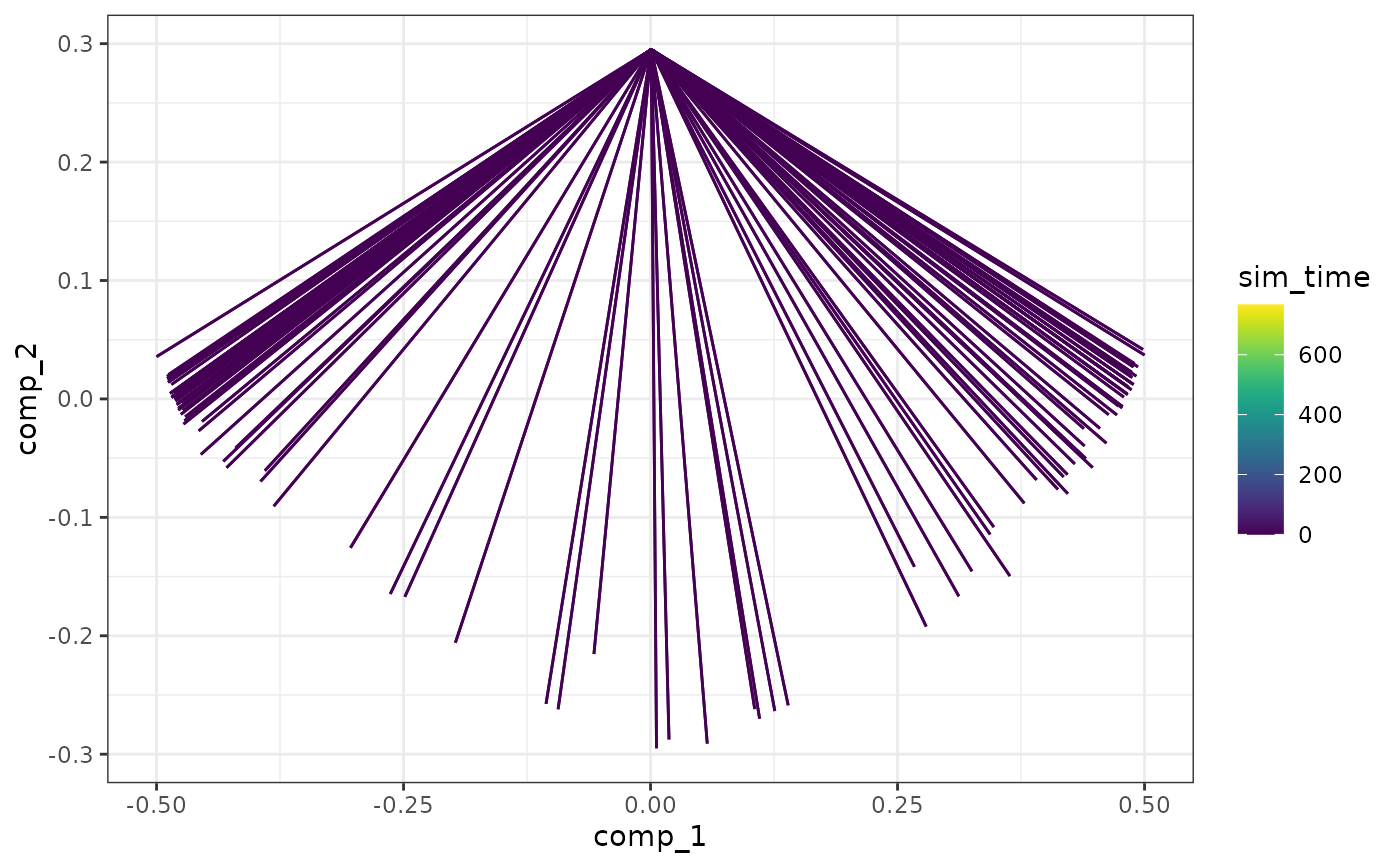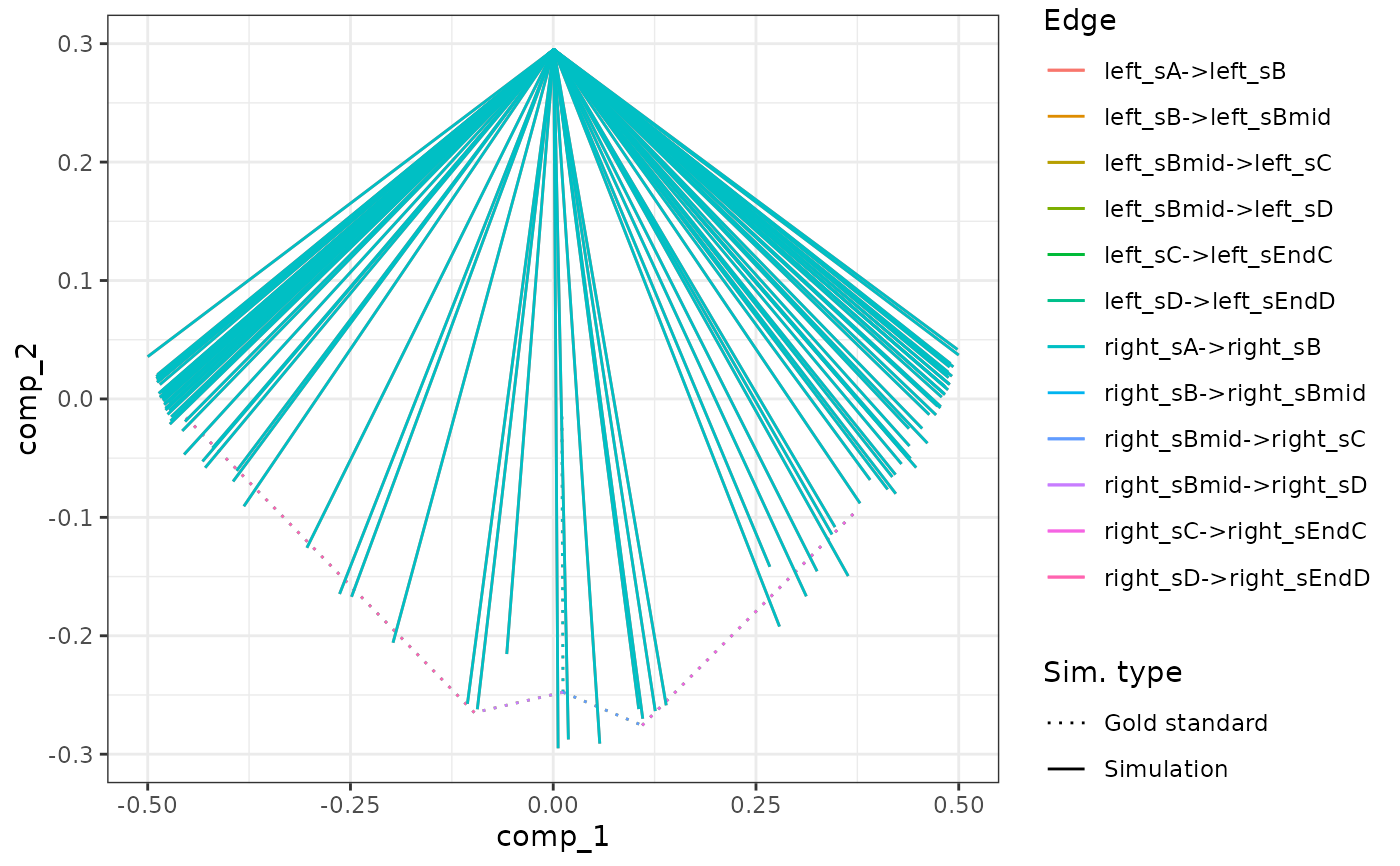Assume the given models have the exact same feature ids and ran up until the generate_cells() step. In addition, the user is expected to run generate_experiment() on the combined models.

combine_models(models, duplicate_gold_standard = TRUE)

## Arguments

models A named list of models. The names of the list will be used to prefix the different cellular states in the combined model. Whether or not the gold standards of the models are different and should be duplicated and prefixed.

## Details

See the vignette on simulating batch effects on how to use this function.

## Examples

# \donttest{
data("example_model")
model_ab <- combine_models(list("left" = example_model, "right" = example_model))

# show a dimensionality reduction
plot_simulations(model_ab)plot_gold_mappings(model_ab, do_facet = FALSE)# }